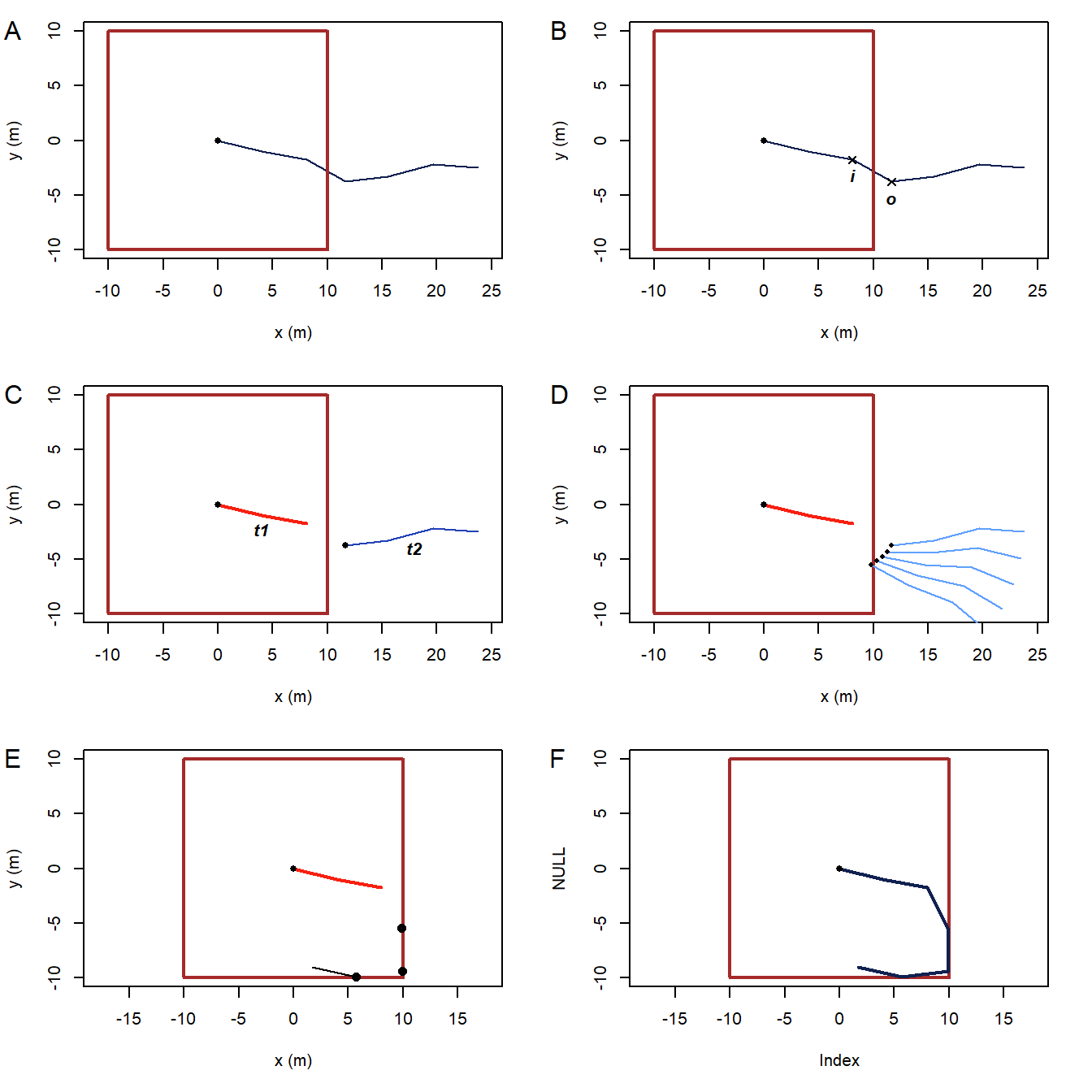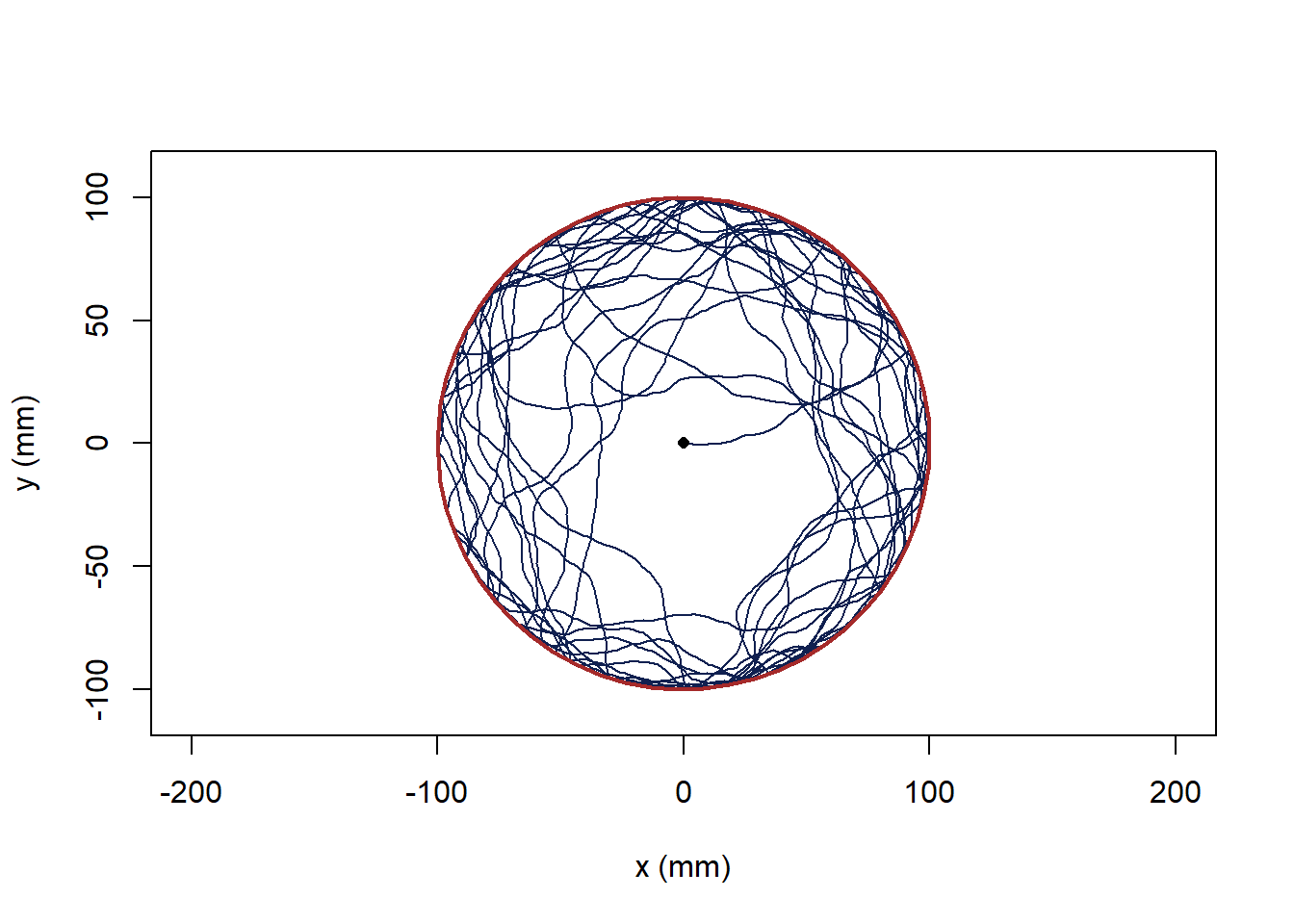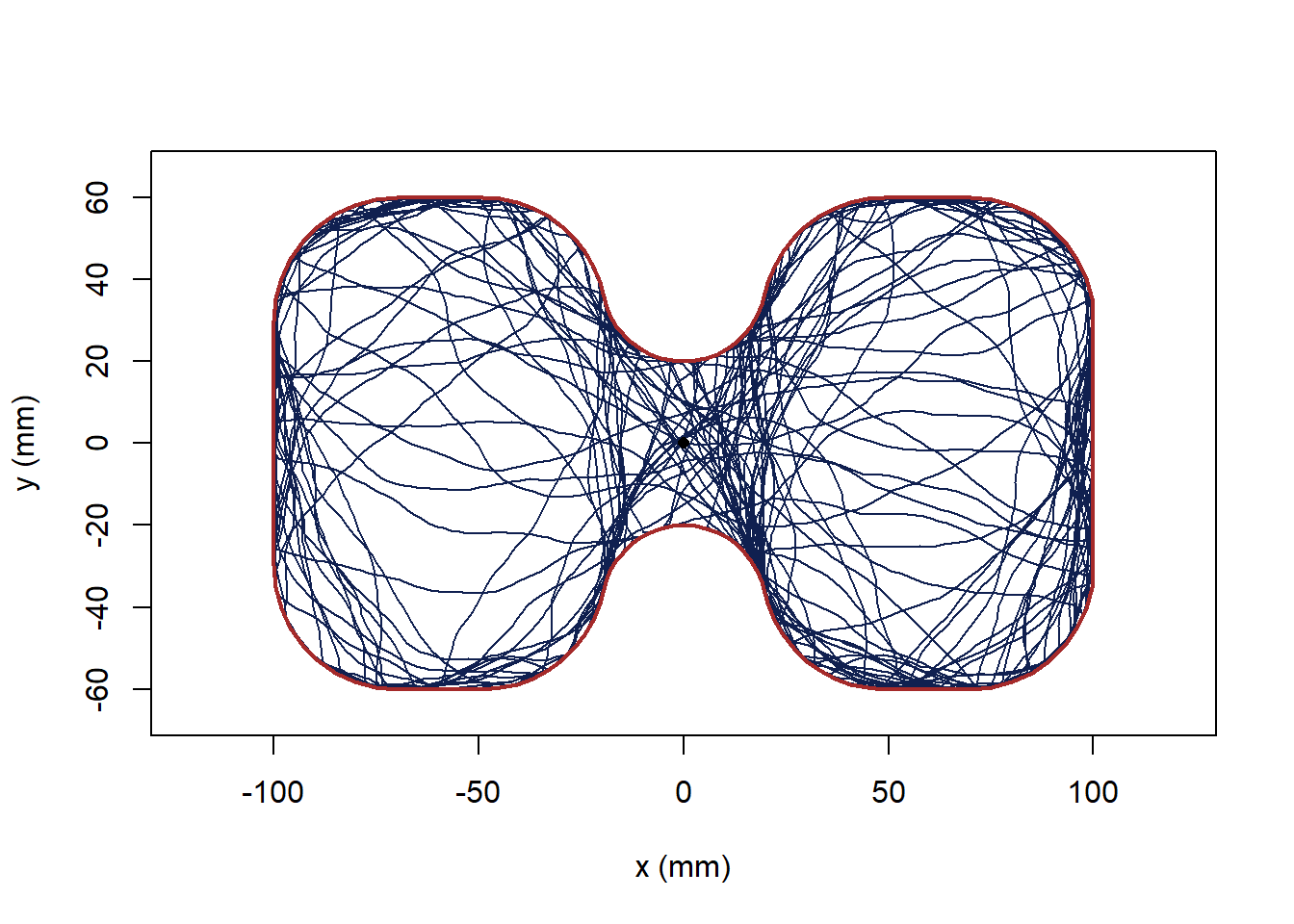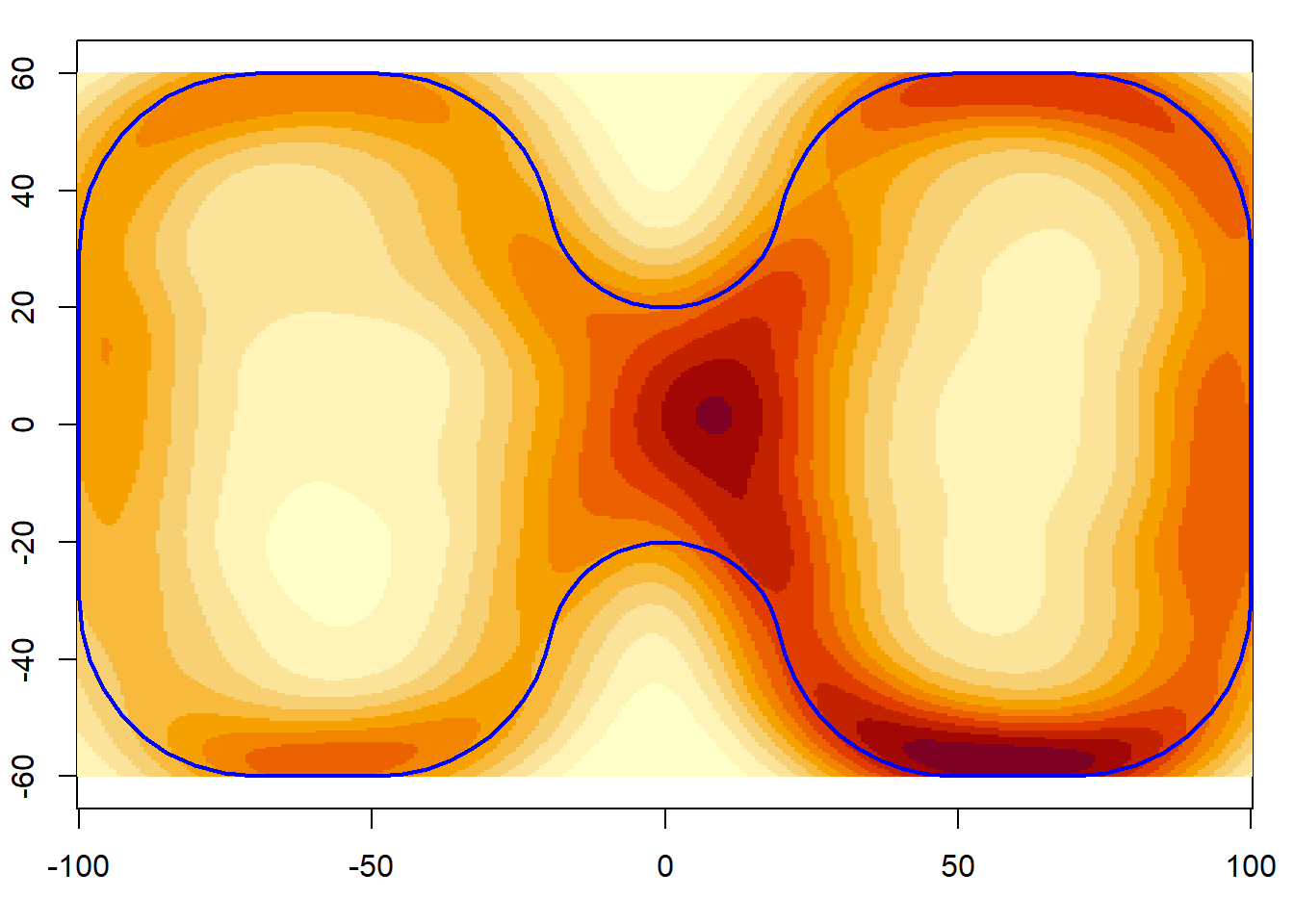## Simulating bounded trajectories

trajr provides some functionality to assist with simulating trajectories. Functions are provided to split and merge trajectories (TrajSplit and TrajMerge), to identify where a trajectory crosses an arbitrary polygon (TrajInPolygon), and to split a trajectory where it first crosses a polygon (TrajSplitAtFirstCrossing). trajr does not attempt to model animal behaviours other than by generating random walks (i.e. the TrajGenerate function). To use the TrajInPolygon or TrajSplitAtFirstCrossing functions, you must have the sp package installed.

To demonstrate one way in which this functionality can be used, I will simulate a trajectory in a bounded space. Let’s assume a very simple behaviour - that animals cannot walk through walls. Whenever a wall is encountered, the animal will turn by the smallest angle possible to allow it to continue walking. This behaviour is not part of the trajr package, however the implementation is fully described in this vignette.

### Our simulation algorithm

To implement the behaviour of not walking through walls, we must define the rules needed to create a constrained trajectory. Obviously, different behaviours would require different rules. The steps for our rules are:

1. Generate a random trajectory that starts inside the boundary (Fig 1A).
2. Find the first point in the trajectory, o, that lies outside the boundary. Let’s name the last point inside the boundary i (Fig 1B).
3. Split the trajectory into two at o: t1 ends at i and lies wholly within the boundary; t2 starts at o, so by definition, t2 starts outside the boundary (Fig 1C). The function TrajSplitAtFirstCrossing performs steps 2 & 3.
4. Determine the minimal rotation $$\alpha$$ of o about i such that o lies within the boundary.
5. Rotate the entire section t2 by $$\alpha$$ (Fig 1D).
6. Go back to step 2 and repeat until all parts of the trajectory lie within the boundary.
7. Merge all the parts back into a single trajectory (Fig 1F). The function TrajMerge performs this operation.Figure 1. Steps to constrain a trajectory following our rules

### The implementation

To start, I will define some general purpose helper functions.

# Some colours
DARKBLUE <- "#102050"
MIDRED <- "#f82010"
MIDBLUE <- "#2040b8"
LIGHTBLUE <- "#60a0ff"

# Return the coordinates of a point in a trajectory as vector. By default, it's the end point.
trjPt <- function(trj, rowIdx = nrow(trj)) {
as.numeric(trj[rowIdx, c(1, 2)])
}

# Rotate pt around origin by angle
rotatePt <- function(origin, pt, angle) {
rm <- matrix(c(cos(angle), sin(angle), -sin(angle), cos(angle)), ncol = 2)
npt <- as.numeric(t(pt) - origin)
rm %*% npt + origin
}

# Generate a dataframe of points that lie along an arc
arcPts <- function(radius = 1, startAngle = 0, endAngle = 2 * pi, cx = 0, cy = 0, numPts = 10) {
angles <- seq(startAngle, endAngle, length.out = numPts)
data.frame(x = cx + radius * cos(angles), y = cy + radius * sin(angles))
}

Here is a function to rotate a trajectory section so its starting point lies inside the boundary. This is the “decision-making” part of the modelled behaviour.

# Rotates trj around origin so that its starting point lies inside the boundary.
# Uses a brute force algorithm to find the minimal rotation: simply tests lots
# of potential rotations.
#
# @param origin The trajectory is rotated around this point.
# @param trj The trajectory to be rotated.
# @param boundary The region to stay inside.
# @param inc Angular increment (in radians) of points to test. The first point
#   tested is inc, then -inc, then 2 * inc, 2 * -inc and so on.
RotateToDeflect <- function(origin, trj, boundary, inc = pi / 90) {
pt2 <- trjPt(trj, 1) # Starting point of trj

# Find a rotation such that pt2 is inside the boundary
angle <- findRotation(origin, pt2, inc)

# Now rotate the whole trajectory around the origin point
TrajRotate(trj, angle, origin = origin, relative = FALSE)
}

# This is the algorithm to find the minimal rotation angle. Simply generates
# lots of angles, then tests them until a suitable angle is found
findRotation <- function(pt1, pt2, inc) {
for (alpha in seq(inc, pi, by = inc)) {
# Rotate pt2 around pt1 by +- alpha
npt2 <- rotatePt(pt1, pt2, alpha)
# point.in.polygon returns 1 if the point is inside
if (sp::point.in.polygon(npt2, npt2, boundary$x, boundary$y) == 1)
return(alpha)
npt2 <- rotatePt(pt1, pt2, -alpha)
if (sp::point.in.polygon(npt2, npt2, boundary$x, boundary$y) == 1)
return(-alpha)
}
stop("Cannot find suitable rotation")
}

Now we will combine all of the work into a single function that adjusts a trajectory so it is constrained to the inside of a boundary.

# Constrains a trajectory to the inside of a boundary, using a simple model of
# behaviour which is: don't walk through walls.
ConstrainTrajectory <- function(trj, boundary) {
# Start adjusting the trajectory so it doesn't cross any walls.
# Find the first crossing, and split into 2 parts
l <- TrajSplitAtFirstCrossing(trj, boundary)

# Now l is a list containing 2 trajectories (which we earlier referred to as t1 & t2).
# Build up a list of rotated parts as we go
parts <- list(l[])
# When l has length 1, the remainder of the trajectory lies inside the boundary
while (length(l) == 2) {

# Rotate the section t2 about the last point in the previous section
t2 <- RotateToDeflect(trjPt(l[]), l[], boundary)

# Work out where the trajectory now crosses the boundary
l <- TrajSplitAtFirstCrossing(t2, boundary)

# Save the rotated section that's inside
parts[[length(parts) + 1]] <- l[]
}

# Put all the parts back together into a single trajectory
TrajMerge(parts)
}

### Running the simulation

Let’s put it all together to simulate an animal walking in a circular arena.

# Circular arena
boundary <- arcPts(100, 0, 2 * pi, numPts = 60)

# Create a random trajectory
set.seed(1)
trj <- TrajGenerate(n = 5000, angularErrorSd = .14, spatialUnits = "mm")

# Constrain it to the arena
constrained <- ConstrainTrajectory(trj, boundary)

plot(constrained, col = DARKBLUE)
polygon(boundary, border = "brown", lwd = 2)Figure 2. Trajectory constrained to a circular arena

Creed & Miller (1990) used an hourglass shaped arena to test for active wall following, i.e. an animal deliberately stays next to the wall while walking. We can use the same shaped arena and a simulated trajectory to see whether random movement (i.e. not actively wall-following) can look like wall-following.

# Build an hourglass-shaped arena similar to Creed & Miller, (1990)
hourglassArena <- function() {
c1 <- arcPts(30, pi, 1.5 * pi, -70, -30)
c2 <- arcPts(30, 1.5 * pi, 1.9 * pi, -49, -30)
c3 <- arcPts(20, .9 * pi, pi / 2, 0, -40)
xs <- c(c1$x, c2$x, c3$x) ys <- c(c1$y, c2$y, c3$y)
# Exploit the 2 axes of symmetry
data.frame(x = c(xs, -rev(xs), -xs, rev(xs)),
y = c(ys, rev(ys), -(ys), -rev(ys)))
}

boundary <- hourglassArena()

# Create a random trajectory
set.seed(2)
trj <- TrajGenerate(n = 10000, stepLength = 2, angularErrorSd = .1, spatialUnits = "mm")

# Constrain it to the arena
constrained <- ConstrainTrajectory(trj, boundary)

plot(constrained, col = DARKBLUE)
polygon(boundary, border = "brown", lwd = 2)Figure 3. Trajectory constrained to an hourglass arena

### Results

If we plot a heatmap of the trajectory, we can visualise locations where the animal spends more time. Darker regions indicate areas visited more frequently, and lighter regions are less visited.

d <- MASS::kde2d(constrained$x, constrained$y, n = 300)
par(mar = c(3, 2, 1, 1) + .1)
image(d, asp = 1)
polygon(boundary, border = "blue", lwd = 2)Figure 4. Heatmap of trajectory constrained to an hourglass arena

Since the darker regions are generally adjacent to the walls, it appears that the trajectory is following the walls, even though we know there is no active wall-following behaviour. A close look at Figure 3. reveals that the trajectory generally leaves the wall at convex bends, suggesting (correctly) that there is no wall following behaviour occurring.